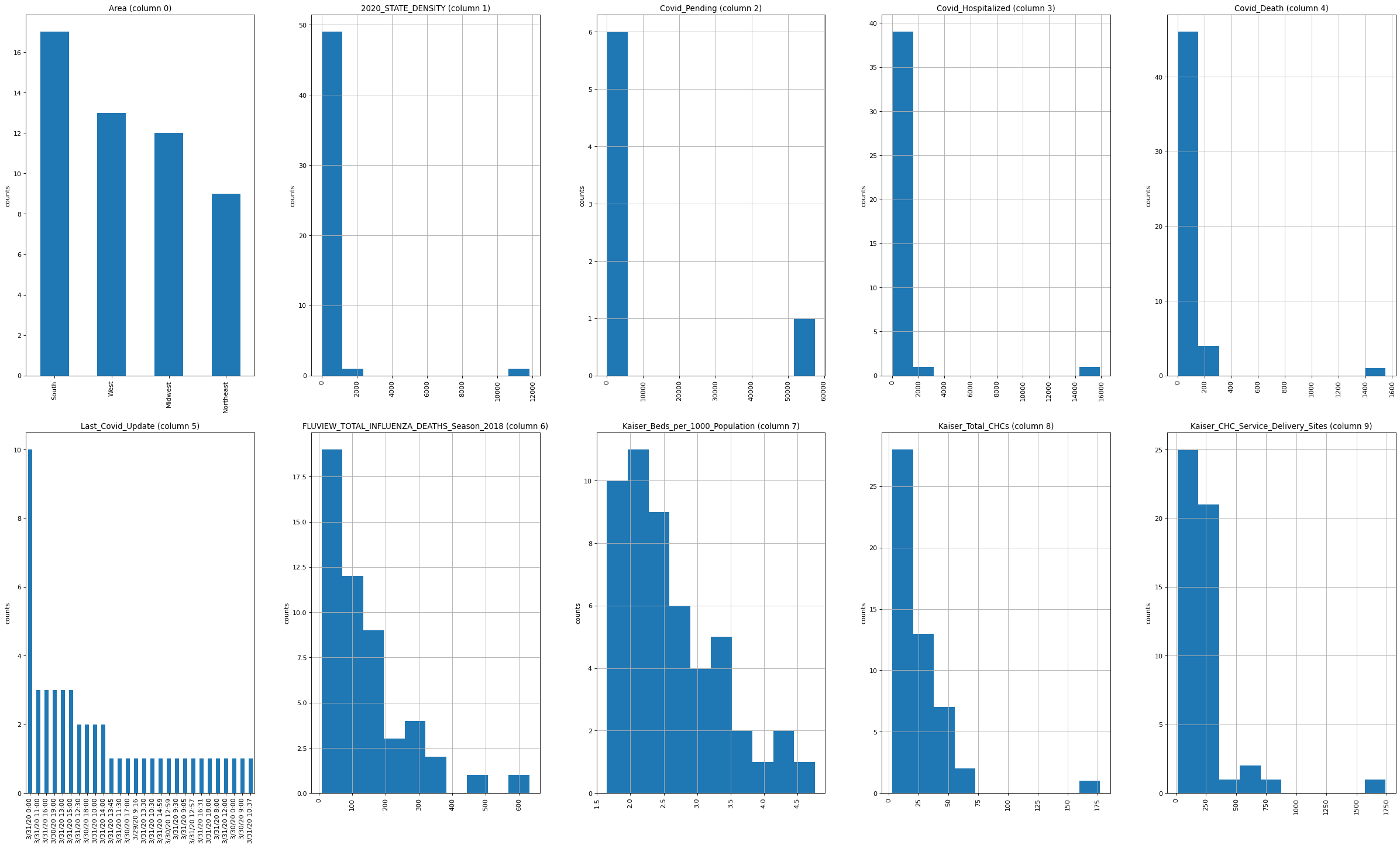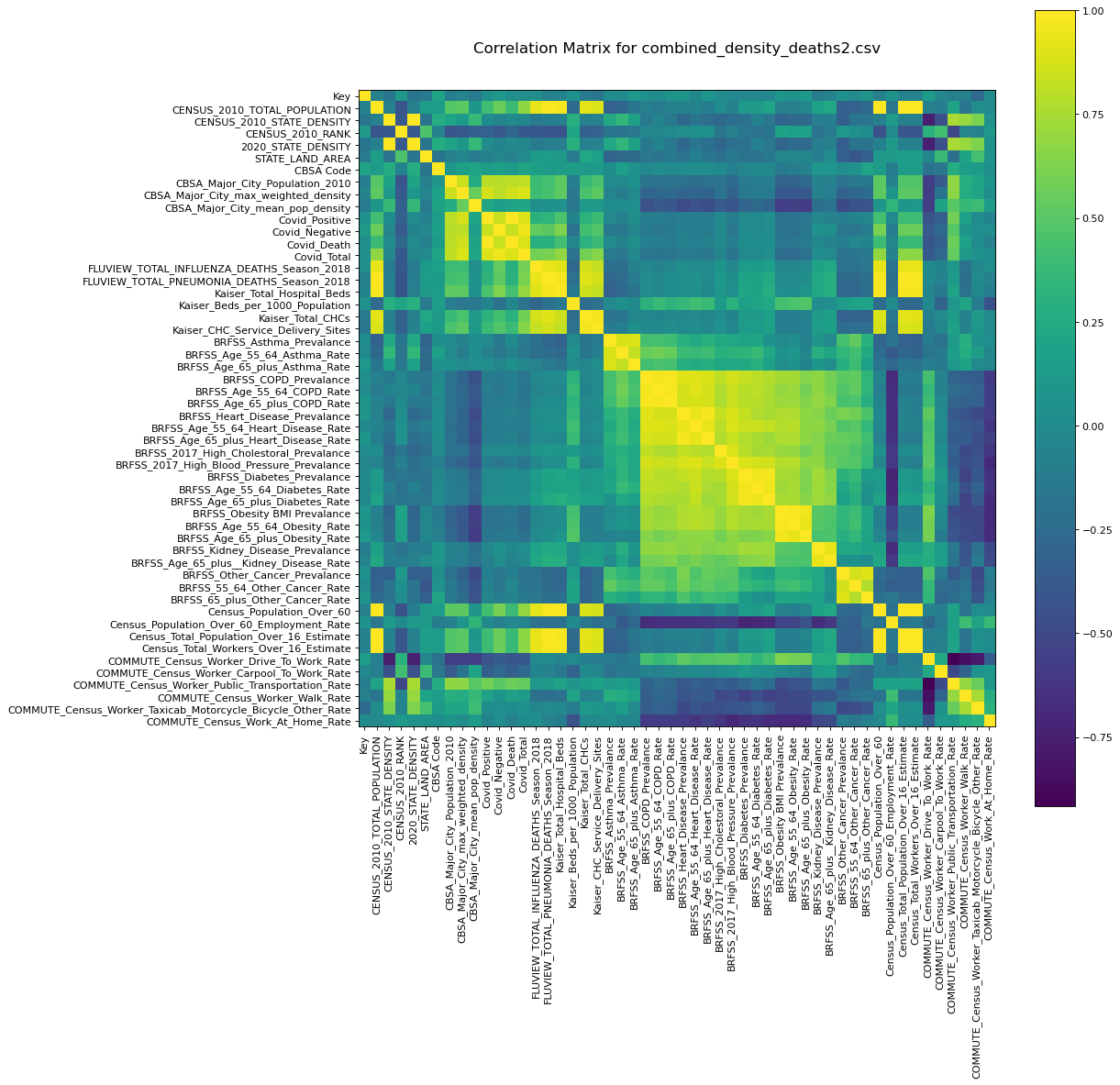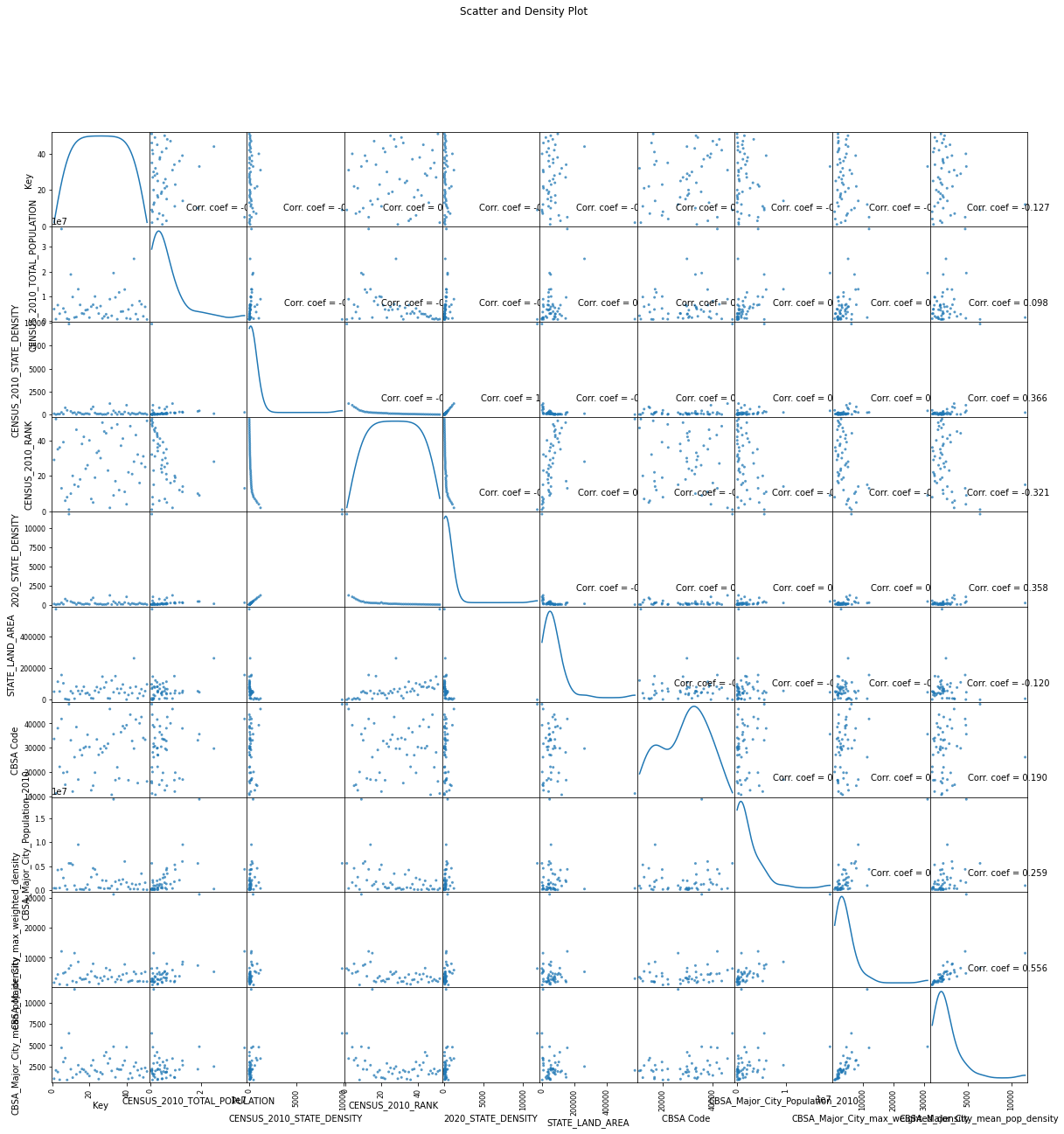## Introduction

Greetings from the Kaggle bot! This is an automatically-generated kernel with starter code demonstrating how to read in the data and begin exploring. If you're inspired to dig deeper, click the blue "Fork Notebook" button at the top of this kernel to begin editing.

## Exploratory Analysis

To begin this exploratory analysis, first import libraries and define functions for plotting the data using matplotlib. Depending on the data, not all plots will be made. (Hey, I'm just a simple kerneling bot, not a Kaggle Competitions Grandmaster!)

from mpl_toolkits.mplot3d import Axes3D
from sklearn.preprocessing import StandardScaler
import matplotlib.pyplot as plt # plotting
import numpy as np # linear algebra
import os # accessing directory structure
import pandas as pd # data processing, CSV file I/O (e.g. pd.read_csv)


There is 1 csv file in the current version of the dataset:

for dirname, _, filenames in os.walk('/kaggle/input'):
for filename in filenames:
print(os.path.join(dirname, filename))

/kaggle/input/combined_density_deaths2.csv


The next hidden code cells define functions for plotting data. Click on the "Code" button in the published kernel to reveal the hidden code.

# Distribution graphs (histogram/bar graph) of column data
def plotPerColumnDistribution(df, nGraphShown, nGraphPerRow):
nunique = df.nunique()
df = df[[col for col in df if nunique[col] > 1 and nunique[col] < 50]] # For displaying purposes, pick columns that have between 1 and 50 unique values
nRow, nCol = df.shape
columnNames = list(df)
nGraphRow = (nCol + nGraphPerRow - 1) / nGraphPerRow
plt.figure(num = None, figsize = (6 * nGraphPerRow, 8 * nGraphRow), dpi = 80, facecolor = 'w', edgecolor = 'k')
for i in range(min(nCol, nGraphShown)):
plt.subplot(nGraphRow, nGraphPerRow, i + 1)
columnDf = df.iloc[:, i]
if (not np.issubdtype(type(columnDf.iloc), np.number)):
valueCounts = columnDf.value_counts()
valueCounts.plot.bar()
else:
columnDf.hist()
plt.ylabel('counts')
plt.xticks(rotation = 90)
plt.title(f'{columnNames[i]} (column {i})')
plt.show()

# Correlation matrix
def plotCorrelationMatrix(df, graphWidth):
filename = df.dataframeName
df = df.dropna('columns') # drop columns with NaN
df = df[[col for col in df if df[col].nunique() > 1]] # keep columns where there are more than 1 unique values
if df.shape < 2:
print(f'No correlation plots shown: The number of non-NaN or constant columns ({df.shape}) is less than 2')
return
corr = df.corr()
plt.figure(num=None, figsize=(graphWidth, graphWidth), dpi=80, facecolor='w', edgecolor='k')
corrMat = plt.matshow(corr, fignum = 1)
plt.xticks(range(len(corr.columns)), corr.columns, rotation=90)
plt.yticks(range(len(corr.columns)), corr.columns)
plt.gca().xaxis.tick_bottom()
plt.colorbar(corrMat)
plt.title(f'Correlation Matrix for {filename}', fontsize=15)
plt.show()

# Scatter and density plots
def plotScatterMatrix(df, plotSize, textSize):
df = df.select_dtypes(include =[np.number]) # keep only numerical columns
# Remove rows and columns that would lead to df being singular
df = df.dropna('columns')
df = df[[col for col in df if df[col].nunique() > 1]] # keep columns where there are more than 1 unique values
columnNames = list(df)
if len(columnNames) > 10: # reduce the number of columns for matrix inversion of kernel density plots
columnNames = columnNames[:10]
df = df[columnNames]
ax = pd.plotting.scatter_matrix(df, alpha=0.75, figsize=[plotSize, plotSize], diagonal='kde')
corrs = df.corr().values
for i, j in zip(*plt.np.triu_indices_from(ax, k = 1)):
ax[i, j].annotate('Corr. coef = %.3f' % corrs[i, j], (0.8, 0.2), xycoords='axes fraction', ha='center', va='center', size=textSize)
plt.suptitle('Scatter and Density Plot')
plt.show()


Now you're ready to read in the data and use the plotting functions to visualize the data.

### Let's check 1st file: /kaggle/input/combined_density_deaths2.csv

nRowsRead = 1000 # specify 'None' if want to read whole file
# combined_density_deaths2.csv may have more rows in reality, but we are only loading/previewing the first 1000 rows
df1.dataframeName = 'combined_density_deaths2.csv'
nRow, nCol = df1.shape
print(f'There are {nRow} rows and {nCol} columns')

There are 51 rows and 65 columns


Let's take a quick look at what the data looks like:

df1.head(5)

REGION Key Area CENSUS_2010_TOTAL_POPULATION CENSUS_2010_STATE_DENSITY CENSUS_2010_RANK 2020_STATE_DENSITY STATE_LAND_AREA Major_City_CBSA_Title CBSA Code ... Census_Population_Over_60 Census_Population_Over_60_Employment_Rate Census_Total_Population_Over_16_Estimate Census_Total_Workers_Over_16_Estimate COMMUTE_Census_Worker_Drive_To_Work_Rate COMMUTE_Census_Worker_Carpool_To_Work_Rate COMMUTE_Census_Worker_Public_Transportation_Rate COMMUTE_Census_Worker_Walk_Rate COMMUTE_Census_Worker_Taxicab_Motorcycle_Bicycle_Other_Rate COMMUTE_Census_Work_At_Home_Rate
0 Alabama 1 South 4779736 94.4 29 97 50645 Mobile, AL Metro Area 33660 ... 1149135 0.24 3854015 2055708 0.86 0.08 0.00 0.01 0.01 0.03
1 Alaska 2 West 710231 1.2 52 1 570641 Anchorage, AK Metro Area 11260 ... 134012 0.35 548676 337552 0.68 0.12 0.01 0.08 0.06 0.05
2 Arizona 3 West 6392017 56.3 35 65 113594 Phoenix-Mesa-Glendale, AZ Metro Area 38060 ... 1695429 0.24 5608516 3155343 0.76 0.11 0.02 0.02 0.03 0.07
3 Arkansas 4 South 2915918 56.0 36 58 52035 Fayetteville-Springdale-Rogers, AR-MO Metro Area 22220 ... 695572 0.25 2336993 1297409 0.83 0.10 0.00 0.02 0.01 0.04
4 California 5 West 37253956 239.1 13 256 155779 San Francisco-Oakland-Fremont, CA Metro Area 41860 ... 7963713 0.29 31085639 18399115 0.74 0.10 0.05 0.03 0.03 0.06

5 rows × 65 columns

Distribution graphs (histogram/bar graph) of sampled columns:

plotPerColumnDistribution(df1, 10, 5)

/opt/conda/lib/python3.6/site-packages/pandas/plotting/_matplotlib/tools.py:307: MatplotlibDeprecationWarning:
The rowNum attribute was deprecated in Matplotlib 3.2 and will be removed two minor releases later. Use ax.get_subplotspec().rowspan.start instead.
layout[ax.rowNum, ax.colNum] = ax.get_visible()
/opt/conda/lib/python3.6/site-packages/pandas/plotting/_matplotlib/tools.py:307: MatplotlibDeprecationWarning:
The colNum attribute was deprecated in Matplotlib 3.2 and will be removed two minor releases later. Use ax.get_subplotspec().colspan.start instead.
layout[ax.rowNum, ax.colNum] = ax.get_visible()
/opt/conda/lib/python3.6/site-packages/pandas/plotting/_matplotlib/tools.py:313: MatplotlibDeprecationWarning:
The rowNum attribute was deprecated in Matplotlib 3.2 and will be removed two minor releases later. Use ax.get_subplotspec().rowspan.start instead.
if not layout[ax.rowNum + 1, ax.colNum]:
/opt/conda/lib/python3.6/site-packages/pandas/plotting/_matplotlib/tools.py:313: MatplotlibDeprecationWarning:
The colNum attribute was deprecated in Matplotlib 3.2 and will be removed two minor releases later. Use ax.get_subplotspec().colspan.start instead.
if not layout[ax.rowNum + 1, ax.colNum]:Correlation matrix:

plotCorrelationMatrix(df1, 14)Scatter and density plots:

plotScatterMatrix(df1, 20, 10)## Conclusion

This concludes your starter analysis! To go forward from here, click the blue "Fork Notebook" button at the top of this kernel. This will create a copy of the code and environment for you to edit. Delete, modify, and add code as you please. Happy Kaggling!